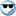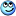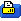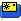May 11, 2005, 08:07 AM
AMA 353531

# Simplex airfoil formula?

Hello, all. I'm looking for a formula that I can use to plot a simplex (logarithmic spiral) airfoil for various cambers in a spreadsheet.

I found a stand-lone plot utility, but I'm a mac user- and I'd rather understand the math a little anyway.

A quick google found lots of references to simplex templates and to logarithmic spirals, but I didn't get anything that related the spiral to camber...
 Sign up now to remove ads between postsMay 11, 2005, 08:42 AM Registered User http://www.modelresearchlabs.com/pricelist.htm Expanding Logarithmic Spiral Patterns Makes tapered wings easy, draws any airfoil from 1% to 10% thick and 1" to 20" chord \$5.00 Example: http://www.nasg.com/afdb/show-airfoil-e.phtml?id=3 A18 airfoil design made with three logarithmic spiral arcs.
 May 11, 2005, 09:04 AM Registered User Also: http://www.mathsoft.com/mathresource...,,1941,00.html "Logarithmic spirals are found throughout nature, for example, the shell of a chambered nautilus, the tusks of an elephant and patterns in sunflowers and pine cones. The Mathcad file logspira.mcd discusses how we obtained the diagram presented earlier and further topics."
May 11, 2005, 09:27 AM
Registered User
Quote:
 A quick google found lots of references to simplex templates and to logarithmic spirals, but I didn't get anything that related the spiral to camber...
I did not have time to check it thoroughly, but I think camber can be calculated thus :
Camber (% chord) = 100 x Sin(A) x e^(-A/tan(A))
where A is the generator angle of the logarithmic spiral, in radians.
If the angle is in degrees, divide it by 57.3
For A = 6�, I find Camber = 3.83 %, which sounds like a good start ?
There remains to figure A from the CamberI started from this formula :
Rho = Chord x e^(Theta/tan(A))
where Rho is the radius, and Theta is the angle of the current radius line.
(Look here for reference : http://www-groups.dcs.st-and.ac.uk/~...uiangular.html)
I would use Theta = -90� as a start point (radius line vertical at the leading edge)
and go to Theta = 0� (radius line horizontal through L.E. and T.E. points).
At each angle step between -90� and 0�, calculate X and Y from Rho and Theta :
X = Rho x Cos(Theta) and Y = Rho x Sin(-Theta)

(The high camber point is where the tangent to the airfoil is horizontal, which occurs when Theta = -A, hence my camber formula above)
Last edited by JMP_blackfoot; May 11, 2005 at 11:00 AM.
 May 11, 2005, 11:13 AM Registered User "This little program creates logarithmic spiral airfoils based on the thickness you choose. Output can be sent to printer or plotter." http://www.smallflyingarts.com/Downloads/Downloads.htm
May 11, 2005, 11:34 AM
Registered User
Rdeis wrote :
Quote:
 I found a stand-lone plot utility, but I'm a mac user- and I'd rather understand the math a little anyway.
Obviously, the download program is the easy way, but it does not help with understanding the mathMay 11, 2005, 01:56 PM
AMA 353531
Quote:
 Originally Posted by Ollie "This little program
That's the one I found- works great on a windows box, but I don't have any windows boxes with print capability. Only my macs are allowed to talk to the printers. (-:

So if I understand you guys correctly, I should be able to use a logarithmic spiral formula to plot (r,theta), and for the correct camber I can solve the eqn "Camber (% chord) = 100 x Sin(A) x e^(-A/tan(A))" for A, then start the spiral plot with theta = A.

Or, perhaps easier, plot the given equation and pick an A off the curve that corresponds to the desired camber.

Right?
May 12, 2005, 12:44 AM
Registered User
Quote:
 Originally Posted by rdeis So if I understand you guys correctly, I should be able to use a logarithmic spiral formula to plot (r,theta), and for the correct camber I can solve the eqn "Camber (% chord) = 100 x Sin(A) x e^(-A/tan(A))" for A, then start the spiral plot with theta = A. Or, perhaps easier, plot the given equation and pick an A off the curve that corresponds to the desired camber. Right?
Yes, that's exactly the idea. Once you've solved the equation for A as a function of Camber, would you let us know ? Thank you in advance.
I think that, given the small angles A involved, it will be possible to use a simpler polynomial approximation for A = f(Camber) and not lose any significant precision. I'll have a look on my side.
 May 12, 2005, 02:51 AM Registered User Approximation function : A (degrees) = 1.554 x Camber(%) is accurate enough. It is accurate within 1% from 0 to over 10% camber. For example : A = 1� -> Camber = 0.6421 %; Approximation = 0.644 %; Relative error = 0.644/0.6421 - 1 = 0.3% A = 7.8� -> Camber = 5.0233 %; Approximation = 5.0232 %; Relative error = 5.0232/5.0233 - 1 = 0 % A = 16� -> Camber = 10.4078 %; Approximation = 10.3040 %; Relative error = 10.3040/10.4078 - 1 = - 1 %; This is good enough for all practical purposes in my opinion. Relative error is nearly zero (<0.2 %) for cambers between 5~10 % Last edited by JMP_blackfoot; May 12, 2005 at 03:03 AM.
 May 16, 2005, 12:42 AM Registered User Rdeis, Any luck with the spreadsheet ?
 May 16, 2005, 11:00 AM AMA 353531 Not yet, got distracted pulling the motor out of my car.. back to you soon.. (-:
May 16, 2005, 11:33 PM
AMA 353531

# Got it..

Excell spreadsheet attached, I can't upload .xls, so I renamed the file with a legal extension. You'll have to rename it with a .xls extension to use it, attachements are itendical in all but name in case the upload protocol depends on name.

It produces airfoil-shaped plots, but I haven't carefully scaled one for printing yet. Chord, Camber, and resolution are set from cells in the upper left.

Interesting tidbit: I have it set for a 10 <insert units> chord. For even camber % numbers, it produces a smooth plot from 0-10. For odd camber numbers, it plots from 5-10 and only gives the back half of the airfoil?
 May 17, 2005, 11:10 AM AMA 353531 The .doc works, I just downloaded it to a WIntel box, renamed it with a .xls extension, and opened it in excell. Neat.
 May 17, 2005, 11:30 AM AMA 353531 Ahah! You can fix the print scaling easily by using hte "print selected plot" feature, measuring the length of the printed axis, then manually setting the X and Y axis scales to go from 0 to the length. After doing that and making two prints I could overlay them and show that a 4" 10% camber plot does indeed match the first half of an 8" 10% camber plot. Fantastic! Thanks for your help, everyone.
May 17, 2005, 11:52 AM
Registered User
rdeis,

I reworked your spreadsheet a little, so as to spread the values better along the X-axis :
- introduced an X0 column between Rho and X, used for calculating Theta intervals
- Theta is calculated so as to spread the intervals in a more practical manner
- Y is modified for calculation with -Theta
It works with any reasonable value of Camber, even fractional.

Hope you like it.

### Files

 Thread ToolsShow Printable VersionEmail this Page